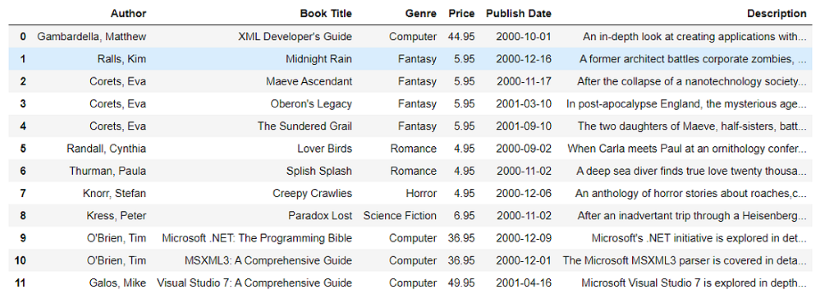GeeksforGeeks App
Open AppBrowser
Continue

# Convert XML structure to DataFrame using BeautifulSoup – Python

Here, we are going to convert the XML structure into a DataFrame using the BeautifulSoup package of Python. It is a python library that is used to scrape web pages. To install this library, the command is

`pip install beautifulsoup4`

We are going to extract the data from an XML file using this library, and then we will convert the extracted data into Dataframe. For converting into the Dataframes, we need to install the panda’s library.

Pandas library: It is a python library which is used for data manipulation and analysis. To install this library, the command is

`pip install pandas`

Note: If it asks you to install a parser library, use the command

`pip install et_xmlfile`

Step-by-step implementation:

Step 1: Import the libraries.

## Python3

 `from` `bs4 ``import` `BeautifulSoup  ``import` `pandas as pd`

First we need to import the libraries which are going to use in our program. Here, we imported the BeautifulSoup library from the bs4 module and also imported the pandas library and created its alias as ‘pd’.

Step 2: Read the xml file.

## Python3

 `file` `=` `open``(``"gfg.xml"``,``'r'``)``contents ``=` `file``.read()`

Here, we are opening our xml file named ‘gfg.xml’ using open(“filename”, “mode”) function in read mode ‘r’ and storing it in variable ‘file’. Then we are reading the actual contents stored in the file using read() function.

Step 3:

## Python3

 `soup ``=` `BeautifulSoup(contents,``'xml'``)`

Here, we are giving the data of the file to be scraped which is stored in the ‘contents’ variable to the BeautifulSoup function and also passing the type of file which is XML.

Step 4: Searching the data.

Here, we are extracting the data. We are using the find_all() function which returns the extracted data present inside the tag which is passed in this function.

## Python3

 `authors ``=` `soup.find_all(``'author'``)``titles ``=` `soup.find_all(``'title'``)``prices ``=` `soup.find_all(``'price'``)``pubdate ``=` `soup.find_all(``'publish_date'``)``genres ``=` `soup.find_all(``'genre'``)``des ``=` `soup.find_all(``'description'``)`

Example:

`authors = soup.find_all('author')`

We are storing the extracted data into the authors variable. This find_all(‘author’) function will extract all the data inside the author tag in the xml file. The data will be stored as a list, i.e. authors is a list of extracted data from all the author tag in that xml file. Same with the other statements.

Step 5: Get text data from xml.

## Python3

 `data ``=` `[]``for` `i ``in` `range``(``0``,``len``(authors)):``   ``rows ``=` `[authors[i].get_text(),titles[i].get_text(),``           ``genres[i].get_text(),prices[i].get_text(),``           ``pubdate[i].get_text(),des[i].get_text()]``   ``data.append(rows)`

Now, we have all the data extracted from the xml file in various lists as per the tags. Now we need to combine all the data related to one book from different lists. So we run a for loop where all the data of a particular book from different lists is stored in one list name ‘rows’ and then each such row is appended in another list named ‘data’.

Step 6: Print the dataframe.

Finally, we have a separated combined data for each book. Now we need to convert this list data into a DataFrame.

## Python3

 `df ``=` `pd.DataFrame(data,columns ``=` `[``'Author'``,``'Book Title'``,``                                  ``'Genre'``,``'Price'``,``'Publish Date'``,``                                  ``'Description'``], dtype ``=` `float``)``display(df)`

Output:DataFrame

Here, we are converting that data list into a Dataframe using the pd.DataFrame() command. In this command we pass the list ‘data’ and also passed the names of the columns we want to have. We have also mentioned the datatype(dtype) as float which will make all the numerical values float.

Now we have extracted the data from the XML file using the BeautifulSoup into the DataFrame and it is stored as ‘df’. To see the DataFrame we use the print statement to print it.

XML File Used – GFG.xml

Below is the full implementation:

## Python3

 `# Python program to convert xml``# structure into dataframes using beautifulsoup`` ` `# Import libraries``from` `bs4 ``import` `BeautifulSoup``import` `pandas as pd`` ` `# Open XML file``file` `=` `open``(``"gfg.xml"``, ``'r'``)`` ` `# Read the contents of that file``contents ``=` `file``.read()`` ` `soup ``=` `BeautifulSoup(contents, ``'xml'``)`` ` `# Extracting the data``authors ``=` `soup.find_all(``'author'``)``titles ``=` `soup.find_all(``'title'``)``prices ``=` `soup.find_all(``'price'``)``pubdate ``=` `soup.find_all(``'publish_date'``)``genres ``=` `soup.find_all(``'genre'``)``des ``=` `soup.find_all(``'description'``)`` ` `data ``=` `[]`` ` `# Loop to store the data in a list named 'data'``for` `i ``in` `range``(``0``, ``len``(authors)):``    ``rows ``=` `[authors[i].get_text(), titles[i].get_text(), genres[i].get_text(``    ``), prices[i].get_text(), pubdate[i].get_text(), des[i].get_text()]``    ``data.append(rows)`` ` `# Converting the list into dataframe``df ``=` `pd.DataFrame(data, columns``=``[``'Author'``,``                                 ``'Book Title'``, ``'Genre'``, ``                                 ``'Price'``, ``'Publish Date'``,``                                 ``'Description'``], dtype ``=` `float``)``display(df)`

Output:DataFrame

My Personal Notes arrow_drop_up